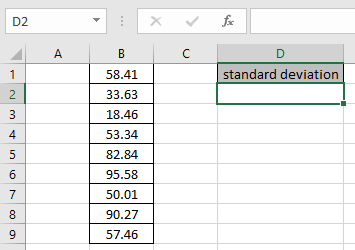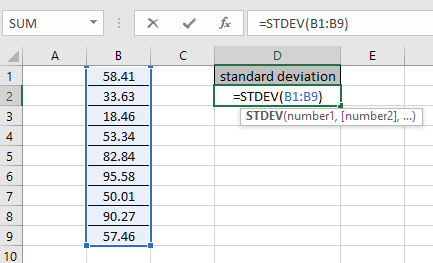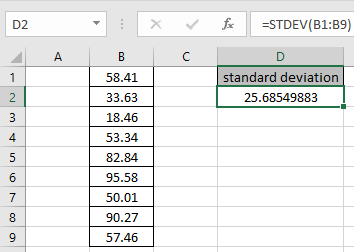# How to Use STDEV Function in Excel

In this article, we will learn about how to use STDEV function in Excel to calculate the standard deviation of the range.

STDEV function is a built-in function to calculate the standard deviation of the range of data provided. There are more versions of this function used for a different set of values.

1. STDEVP: This function used for data set having population
2. STDEV.S: This function used for data set have sample values
3. STDEV.P: This function is newer version for STDEVP. Excel recommends this over STDEVP
4. STDEVA: This function accepts the text or cell reference values which other STDEV functions ignore.
5. STDEVPA: This function accepts population as data set and text and logic_values.

Syntax of STDEV function:

=STDEV (number1, [number2], ...)

Let’s understand this function using it an example.Here we have a range of values from B1:B9 cell.
We just need to use the formula

=STDEV(B1:B9)Using the above formula in the cell to get the standard deviation of the values.The Standard deviation of the range is 25.685.

Hope you understood how to use STDEV function to calculate standard deviation in Excel. Explore more articles on Statistical Excel function here. Please feel free to state your query or feedback for the above article.

Popular Articles:

50 Excel Shortcuts to Increase Your Productivity

How to use the VLOOKUP Function in Excel

How to use the COUNTIF function in Excel 2016

How to Use SUMIF Function in Excel

Terms and Conditions of use

The applications/code on this site are distributed as is and without warranties or liability. In no event shall the owner of the copyrights, or the authors of the applications/code be liable for any loss of profit, any problems or any damage resulting from the use or evaluation of the applications/code.